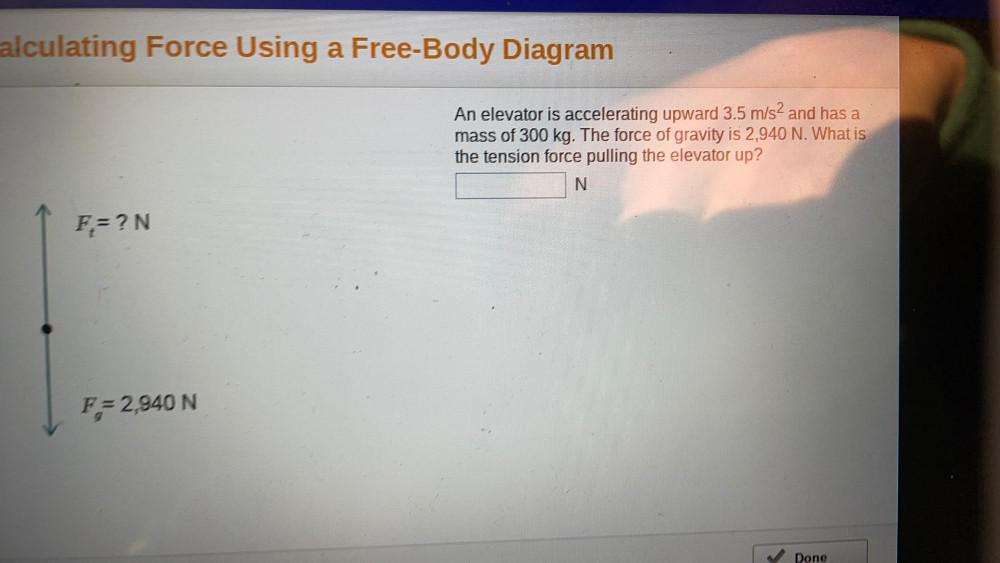Question:

# An elevator is accelerating upward 3.5 m/s² and has a mass of 300 kg. The force of gravity is 2.900 N. What the tension force pulling the elevator ?An elevator is accelerating upward 3.5 m/s² and has a mass of 300 kg. The force of gravity is 2.900 N. What the tension force pulling the elevator ?